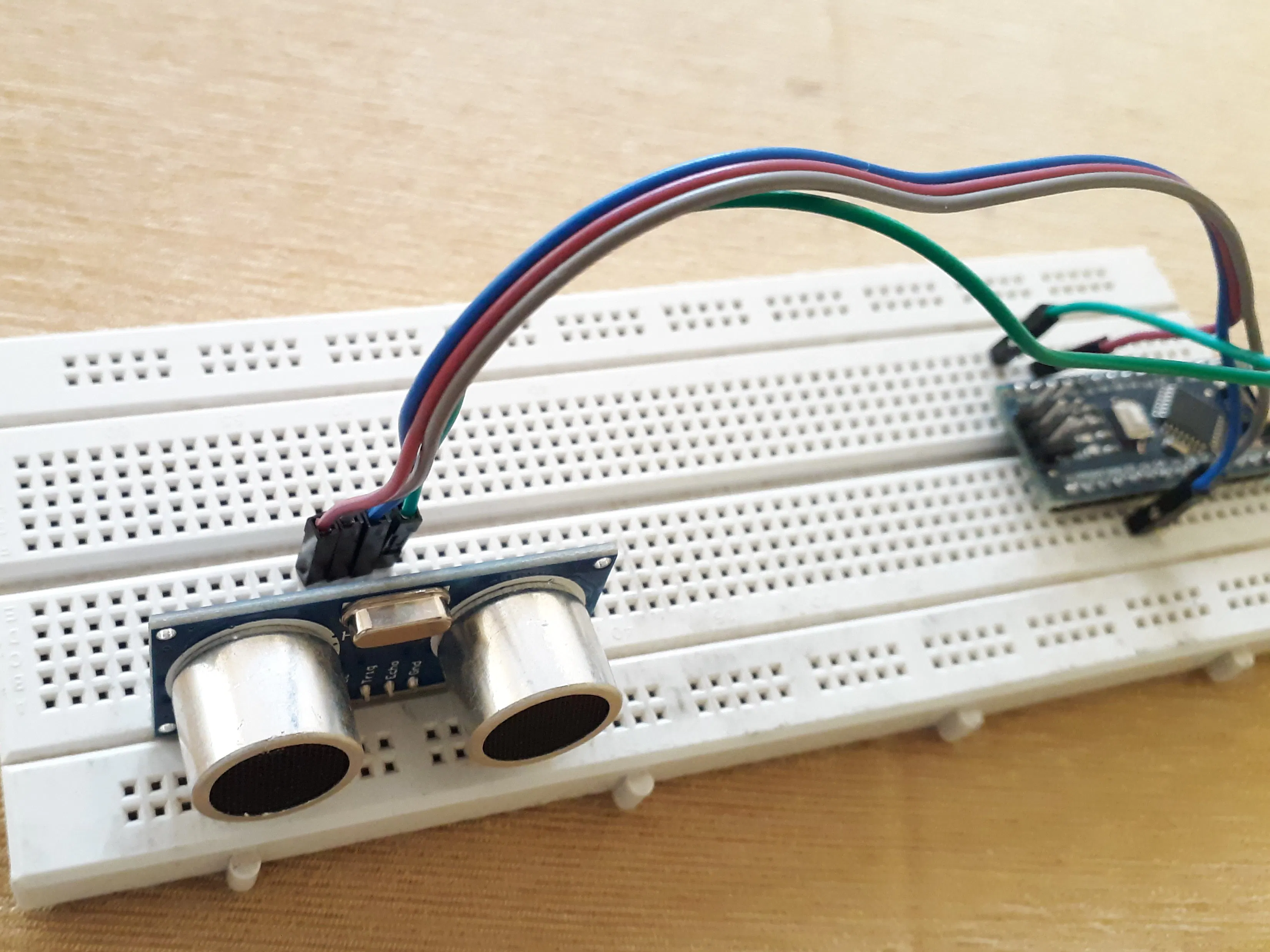# Ultra sonic distance finder with live status

In this project, we will make a ultrasonic sensor based distance finder, but with live status, that is - object coming or going far.

BeginnerFull instructions provided801## Things used in this project

### Hardware componentsUltrasonic Sensor - HC-SR04 (Generic)
×1Arduino Nano R3
×1Jumper wires (generic)
×1×1

## Code

### source code

C/C++
This is source code
```// ---------------------------------------------------------------- //
// made by rho sigma electronics
// Arduino Ultrasoninc Sensor HC-SR04
// Using Arduino IDE 1.8.7
// Using HC-SR04 Module
// ---------------------------------------------------------------- //

#define echoPin 2 // attach pin D2 Arduino to pin Echo of HC-SR04
#define trigPin 3 //attach pin D3 Arduino to pin Trig of HC-SR04

// defines variables
long duration; // variable for the duration of sound wave travel
float distance, distance1, diff;//variable forthedistancemeasuremenorother
int i=0;

void setup() {
pinMode(trigPin, OUTPUT); // Sets the trigPin as an OUTPUT
pinMode(echoPin, INPUT); // Sets the echoPin as an INPUT
Serial.begin(9600); // // Serial Communication is starting with 9600 of baudrate speed
Serial.println("Ultrasonic Sensor HC-SR04 Test"); // print some text in Serial Monitor
Serial.println("with Arduino");
}
void loop() {
// Clears the trigPin condition
digitalWrite(trigPin, LOW);
delayMicroseconds(2);
// Sets the trigPin HIGH (ACTIVE) for 10 microseconds
digitalWrite(trigPin, HIGH);
delayMicroseconds(10);
digitalWrite(trigPin, LOW);
// Reads the echoPin, returns the sound wave travel time in microseconds
duration = pulseIn(echoPin, HIGH);
// Calculating the distance
distance = duration * 0.034 / 2; // Speed of sound wave divided by 2 (go and back)
// Displays the distance on the Serial Monitor
Serial.print("Distance: ");
Serial.print(distance);
Serial.println(" cm");

if(distance <= 5.0){
Serial.println("  object is near");
}
if(distance >= 100.0){
Serial.println("  object is far");
}
if((distance < 100.0) && (distance >5.0)){
Serial.println("  object is in green zone");
}

if(i != 0)
{
diff=abs(distance-distance1);

if(diff > 0.4) //because sensor has an eorrer of 0.3 cm
{
if(distance < distance1)
Serial.println("  object is coming closer");
else
{
if(distance > distance1)
Serial.println("  object is going farther");
else
Serial.println("  object is not moving");
}
}
else
Serial.println("  object is not moving"); //print object is not moving
}

distance1=distance;
i=i+1;

delay(1000); // delay 1 second
}
```

## Credits

### rhosigmaelectronics

1 project • 1 follower